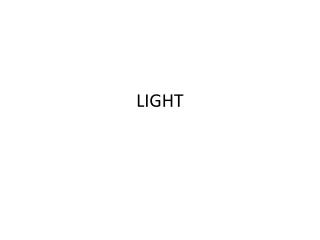DownloadDownload PresentationLIGHT

# LIGHT

Télécharger la présentation## LIGHT

- - - - - - - - - - - - - - - - - - - - - - - - - - - E N D - - - - - - - - - - - - - - - - - - - - - - - - - - -
##### Presentation Transcript

1. LIGHT

2. Refraction • Refraction Fact #1: As light goes from one medium to another, the velocity CHANGES! • Refraction Fact #2: As light goes from one medium to another, the path CHANGES!

3. Refraction – Going from Air to Water • If a light ray goes from a LOW refractive Index (FAST MEDIUM) to a HIGH refractive index (SLOW MEDIUM), it’s speed DECREASES and the angle BENDS TOWARDS the normal

4. Refraction – Going from Water into Air • If you are going from a HIGH refractive index to a LOW refractive index, your speed INCREASES and the angle BENDS AWAY the normal

5. There is one exception… • That is when light hits ‘head-on’, perpendicular to the boundary. The light does not bend but its speed still changes.

6. Total internal reflection • happens when the angle between the light ray and the normal is greater than the critical angle. • The critical angle is formed when the light ray travels along the boundary between the two substances. • Optical fibres use multiple total internal reflections to transmit light.

7. Copy the diagrams into your workbook. • Draw a normal wherever the light rays enter a new substance. • Demonstrate what will happen to the rays as they enter and exit from the substances by continuing the ray through the shape and out the other side.

8. Apparent Depth Prac • Coin and Fish

9. Why does it look bent away from normal and not towards?? • Light from the lower part of the ruler is travelling into a region of lower optical density (air), and so has been bent away from the normal. • The image in the water is actually an illusion—an image our brain constructs based on where light from the lower part of the ruler appears to come from. • It assumes that the light travelled in a perfectly straight line, even though it didn’t.

10. Part B: Refraction in water - ANSWERS • 1 a Refract; towards. • b Emerge; away from. • c Incident; a glass surface; no. • 2 When light rays at an angle to the surface travel from a medium of low density to one of higher density, the light rays refract towards the normal. • 3 a From the tip, to the eye. • 4 a From the coin to the eye.

11. Lenses • There are two main types of lenses: • convex lenses—these curve outwards and are fatter in the middle • concave lenses—these curve inwards (a little like a cave) and are thinner in the middle. Convex lenses Concave Lenses

12. Lenses – An application of refraction • There are 2 basic types of lenses A diverging lens (concave) takes light rays and spreads them outward. A converging lens (Convex) takes light rays and bring them to a point.

13. Movement of Light through Lenses CONVEX LENS • In a convex lens, an incoming ray parallel to the principal axis is refracted through the principal focus (F).

14. Concave Lens • In a concave lens, an incoming ray parallel to the principal axis is refracted so that it appears to come from the principal focus (F).

15. Movement of Light through a Lens • The distance from the centre line (plane) of the lens to the principal focus is called the focal length of the lens. • A ray passing through the centre of either type of lens is unaffected. • As with all images, rays of light that come from a part of the object come together again at that same part of the image.

16. Focal Length • The greater the curvature of a lens, the more it bends light and hence the shorter the focal length.

17. Image type and Location • Convex lenses produce two different types of images, depending on where the object is located. • If the object is at a distance greater than the focal length of the lens, a real image is formed. • A real image can be projected onto a screen

18. Convex Lenses • If the object is at a distance less than the focal length of the lens, a virtual image is formed. • This image can’t be projected onto a screen.

19. Concave Lenses • Concave lenses produce only virtual images.

20. Finding the focal length • Rays coming into a lens from a distant object are almost parallel and form an image very close to the focus. • We can then measure the distance from lens to image to determine the focal length of the lens.

21. Images in a Convex and Concave Lens Prac • AIM: To investigate the image formed by different convex lens and concave lens • Complete Convex prac – using 2 different convex lenses

22. Questions • CONVEX – 1) What happened as the object was brought closer to the lens? 2) When does; • a a real image (on a screen) is obtained • b a virtual image (one that cannot be ‘caught’ on a • screen) is obtained • c no image is obtained • CONCAVE - 1) Assess whether it is possible to form a real image (one that may be ‘caught’ on a screen) using a concave lens. 2) Explain how the image changes as the object-to-lens distance is varied.